# SQL COUNT() function

## COUNT() function

The SQL COUNT() function returns the number of rows in a table satisfying the criteria specified in the WHERE clause. It sets the number of rows or non NULL column values.
COUNT() returns 0 if there were no matching rows.

Syntax:

```COUNT(*)
COUNT( [ALL|DISTINCT] expression )
```

The above syntax is the general SQL 2003 ANSI standard syntax. This helps to understand the way SQL COUNT() Function is used. But different database vendors may have different ways of applying COUNT() function.

Bellow, you can see that MySQL, PostgreSQL, and Microsoft SQL Server follows the same syntax as given above. But DB2 and Oracle differs slightly.

Overall, you can use * or ALL or DISTINCT or some expression along with COUNT to COUNT the number of rows w.r.t. some condition or all of the rows, depending up on the arguments you are using along with COUNT() function.

DBMS Support: COUNT() function

 DBMS Command MySQL Supported PostgreSQL Supported SQL Server Supported Oracle Supported

DB2 and Oracle Syntax:

```COUNT ({*|[DISTINCT] expression}) OVER (window_clause)
```

Parameters:

Name Description
ALL Applies to all values. ALL returns the number of non NULL values.
DISTINCT Ignored duplicate values and COUNT returns the number of unique nonnull values.
expression Expression made up of a single constant, variable, scalar function, or column name and can also be the pieces of a SQL query that compare values against other values. Expression of any type except text or image. Aggregate functions and subqueries are not permitted.
* COUNTs all the rows in the target table whether or not they include NULLs.

Syntax diagram - COUNT() function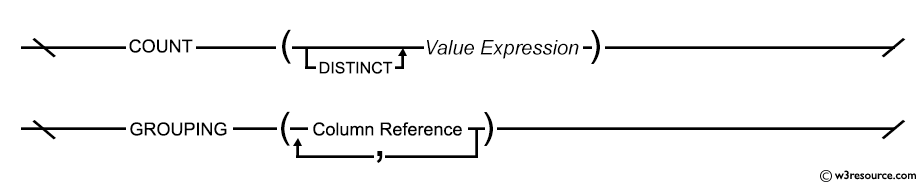In the subsequent pages, we have discussed how to apply COUNT() with various SQL clauses. For those applications, we have used Oracle 10g Express Edition.

An important thing about COUNT() function:

When the * is used for COUNT(), all records ( rows ) are COUNTed if some content NULL but COUNT(column_name) does not COUNT a record if its field is NULL. See the following examples:

## SQL COUNT rows in a table

In the following example, an asterisk character ( * ) is used followed by the SQL COUNT() which indicates all the rows of the table even if there is any NULL value.

Sample table: orders

To get number of rows in the 'orders' table, the following SQL statement can be used:

SQL Code:

``````SELECT COUNT(*)
FROM orders;
``````

Relational Algebra Expression: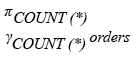Relational Algebra Tree: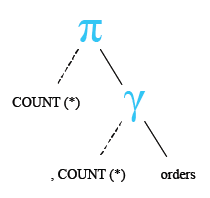Output:

``` COUNT(*)
---------
34
```

Pictorial Presentation: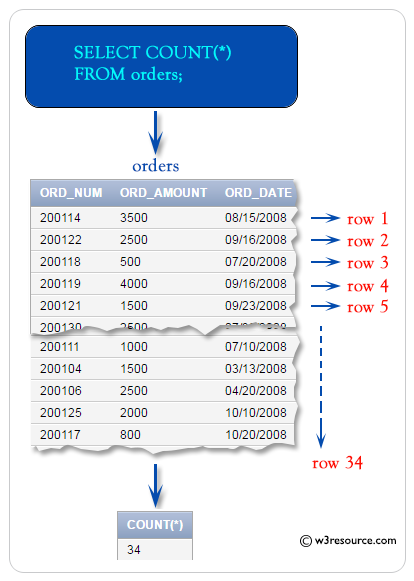## Select COUNT(*) from multiple tables

The following query COUNT the number of rows from two different tables (here we use employees and departments) using COUNT(*) command.

SQL Code:

``````SELECT(
SELECT COUNT(*)
FROM   employees
) AS Total_Employees,
(SELECT COUNT(*)
FROM   departments
) AS No_Of_Departments
FROM dual
``````

Output:

```TOTAL_EMPLOYEES NO_OF_DEPARTMENTS
--------------- -----------------
107                27
```

## SQL COUNT( ) with column name

In this example SQL COUNT() function excludes the NULL values for a specific column if specified the column as an argument in the parenthesis of COUNT function.

Sample table: listofitem

To get the number of rows in the 'listofitem' table with the following condition -

1. COUNT number of rows for the column 'coname'

the following SQL statement can be used :

SQL Code:

``````SELECT COUNT(coname)
FROM listofitem;
``````

Relational Algebra Expression: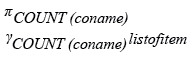Relational Algebra Tree: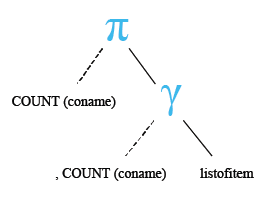Output:

```COUNT(CONAME)
-------------
2
```

Explain:

The above statement COUNTs those rows for the 'coname' column which are not NULL.

## SQL COUNT rows with user defined column heading

To get number of rows in the 'orders' table with the following condition -

1. result have to display with a heading 'Number of Rows',

the following SQL statement can be used:

SQL Code:

``````SELECT COUNT( *) as "Number of Rows"
FROM orders;
``````

Output:

```Number of Rows
--------------
36
```

## SQL COUNT( ) with where clause

The WHERE clause can be used along with SQL COUNT() function to select specific records from a table against a given condition.

Example:

Sample table: orders

To get number of rows in the 'orders' table with following condition -

1. ord_amount against the order is more than 1500,

the following SQL statement can be used :

``````SELECT COUNT( * ) as "Number of Rows"
FROM orders
WHERE ord_amount>1500;
``````

Output:

```Number of Rows
--------------
22
```

## Application of COUNT() function

In the subsequent pages, we have discussed how to apply COUNT() with various SQL clauses. For those applications, we have used Oracle 10g Express Edition.

COUNT with DISTINCT page discusses how to apply COUNT function with DISTINCT and also discusses how to apply COUNT function with ALL clause. Unlike using *, when ALL is used, NULL values are not selected.

COUNT HAVING page discusses how to apply COUNT function with HAVING clause and HAVING and GROUP BY .

COUNT with GROUP BY page discusses how to apply COUNT function with GROUP BY in ascending order and in descending order.

Note: Outputs of the said SQL statement shown here is taken by using Oracle Database 10g Express Edition

Here is a slide presentation of all aggregate functions.

Check out our 1000+ SQL Exercises with solution and explanation to improve your skills.

Previous: Aggregate functions
Next: COUNT with Distinct

﻿

## SQL: Tips of the Day

What's the difference between VARCHAR and CHAR?

VARCHAR is variable-length.

CHAR is fixed length.

If your content is a fixed size, you'll get better performance with CHAR.

Ref: https://bit.ly/3wl3ram

We are closing our Disqus commenting system for some maintenanace issues. You may write to us at reach[at]yahoo[dot]com or visit us at Facebook